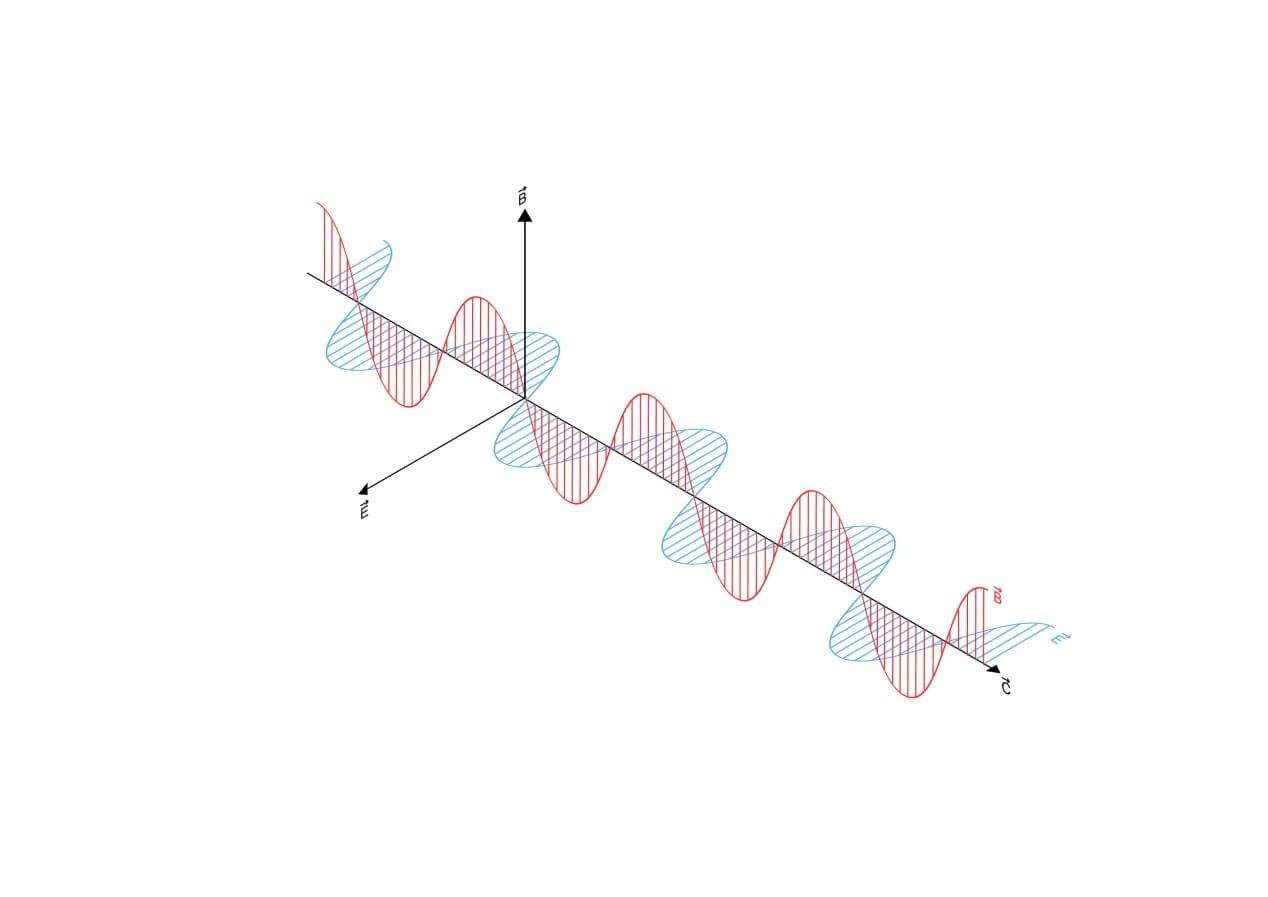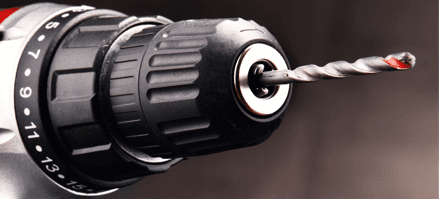## Lesson 9 – Light Waves

Posted on: Friday, April 15th, 2022  In: Learning Optics with Austin

In “What is Light?”, we described light as both a wave and a particle under the conditions of the wave-particle duality. In the previous posts, we have mostly modeled light as a ray when discussing reflection and refraction. Only when we talk about diffraction and wavelength do we mention the wave property of light. While the wave property of light involves more technical knowledge, it answers the question of why white light emerges from a prism as a rainbow.Transverse Wave Diagram

To start, light travels as a transverse wave, meaning that its direction of vibration is perpendicular to the direction of propagation. While we indicate the direction of propagation with an arrow, light is in fact oscillating perpendicular to the direction of propagation. In discussing the oscillation aspect, we go back to concepts of frequency, wavelength, and the index of refraction (n) mentioned in “How to Describe Light” and “How Does Light Travel?”.Drill Bit

A good analogy of the relationship between these three variables is a drill bit. Let the spiraling motion of the drill bit remain constant which is analogous to the frequency of light. The speed at which the bit advances through a particular substance is analogous to the speed at which light travels. So if the bit starts spiraling in air and makes contact with wood, it encounters resistance and moves forward at a slower speed, even as its spiraling speed remains constant. This is analogous to light entering glass where the speed of light slows down but the frequency remains the same.Frequency Equation

For 2 different frequencies of light, their speed in a vacuum is the same, just as if we had 2 drill bits with different spiraling speeds, but traveling forward at the same speed. To travel forward at the same speed, the slower bit would have to travel more distance forward in one turn. Therefore, the faster the speed of the drill, the greater the resistance, and the greater the forward speed change. For light, the higher the frequency of light, the greater the influence of the medium and the slower the forward speed in the medium.Prism and Rainbow

The different forward speeds of light when traveling through a medium explains why white light breaks into a rainbow after traveling through a prism. Since the colors of light have different wavelengths (and thus frequencies), this causes colors with lower frequencies (red) to travel faster than colors with higher frequencies (violet). A white beam of light thus breaks apart into the different colors- a more detailed explanation than white light refracts when traveling through a prism.

Next: Lesson 10 – Interference